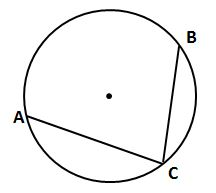# Holt McDougal Larson Geometry: Online Textbook Help Final Exam

Free Practice Test Instructions:

Choose your answer to the question and click 'Continue' to see how you did. Then click 'Next Question' to answer the next question. When you have completed the free practice test, click 'View Results' to see your results. Good luck!

#### Question 5 5. What is the volume of this prism?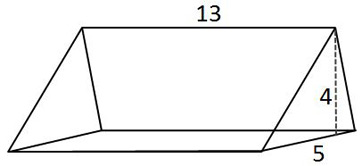#### Question 6 6. In the picture below, if arc CE is 172 degrees and arc BD is 80 degrees, what is the measure of angle CAE?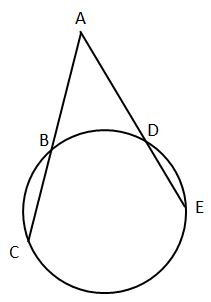#### Question 7 7. In the figure shown below, the graph of the yellow function is a translation of the graph of the blue function. If the expression for the blue function is y(x) = b(x), which of the following is the equation of the yellow function?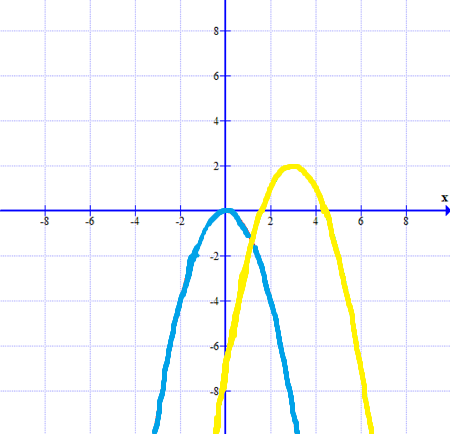#### Question 8 8. In the picture below, if major arc BC is 258 degrees and minor arc BC is 102 degrees, what is the measure of angle BAC?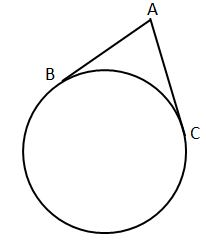#### Question 10 10. How can we explain that the pictured triangles are congruent?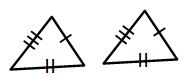#### Question 13 13. If arc AC is 106 degrees and arc BC is 82 degrees, what is the measure of angle ACB?